• Difficulty Level : Basic
• Last Updated : 21 Feb, 2022

A quadrilateral is a 2 – D shape which contain 4 sides. It consist of four sides, four angles and four vertices.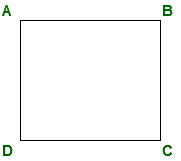Different type of quadrilateral and their properties:

• Rectangle:
The rectangle, like the square, is one of the most commonly known quadrilaterals. It is defined as having all four interior angles 90° (right angles).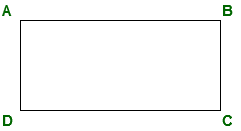• All four angles in a rectangle are 90°.

• Opposite side are parallel and of same length(or congruent).

• Diagonals of rectangle bisect each other and divide a the rectangle into two congruent triangles.

• Area of rectangle = length * breadth.

• Perimeter of rectangle = 2*(length + breadth)
• Square:
In geometry, a square is a regular quadrilateral, which means that it has four equal sides and four equal angles (90-degree angles, or (100-gradian angles or right angles). It can also be defined as a rectangle in which two adjacent sides have equal length.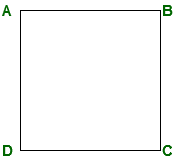• All four angles in a rectangle are 90°.

• All sides are equal and opposite sides are parallel(or congruent).

• Diagonals of square bisect each other at 90° and divide the square into two congruent triangle.

• Area of square = side * side

• Perimeter of square = 4 * side
• Rhombus:
A Rhombus is a flat shape with 4 equal straight sides. A rhombus looks like a diamond. All sides have equal length. Opposite sides are parallel, and opposite angles are equal (it is a Parallelogram).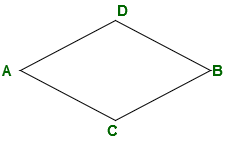• All sides are equal in a rhombus.

• Opposite angles are equal in rhombus.

• The diagonals of rhombus intersect each other at equal angles.

• Area of rhombus = (diagonal1 * diagonal2) / 2

• Perimeter of rhombus = 4 * side
• Trapezium:
In Euclidean geometry, a convex quadrilateral with at least one pair of parallel sides is referred to as a trapezoid.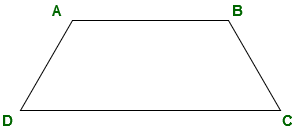• Atleast one pair of opposite side is parallel to each other.

• Area of trapezium = (sum of length of parallel side(or bases)) * height / 2.

• Perimeter of trapezium = sum of all sides.

• Parallelogram:
In Euclidean geometry, a parallelogram is a simple quadrilateral with two pairs of parallel sides. The opposite or facing sides of a parallelogram are of equal length and the opposite angles of a parallelogram are of equal measure.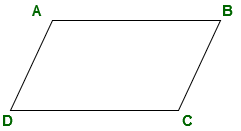• Opposite sides are parallel and congruent to each other.

• Opposite angles are congruent to each other.

• Sum of adjacent angles is 180°

• Diagonals of parallelogram bisect each other and divide the parallelogram into two congruent triangles.

• Area of parallelogram = length * breadth

• Perimeter of parallelogram = 2(length + breadth)

What will be the area and perimeter of given rectangle if length of rectangle is 5cm and breadth of rectangle is 7cm?Solution:

```Area of rectangle = length * breadth.
Area of rectangle = 5 * 7 = 35 cm2
Perimeter of rectangle = 2*(length + breadth)
Perimeter of rectangle = 2*(5 + 7) = 24cm```

What will be the area of given rhombus if diagonal of rhombus are 4cm and 6cm?```Area of rhombus = (diagonal1 * diagonal2) / 2
Area of rhombus = (4 * 6) / 2 = 12 cm2```

What will be area and perimeter of given trapezium if AB is parallel to CD?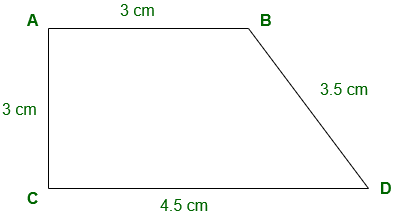```Area of trapezium = (sum of length of parallel side(or bases)) * height / 2.
Area of trapezium = (3 + 4. 5) * 3 / 2
= 11.25 cm2
Perimeter of trapezium = Sum of all sides
= 3 + 3 + 3.5 + 4.5 = 14 cm```
My Personal Notes arrow_drop_up